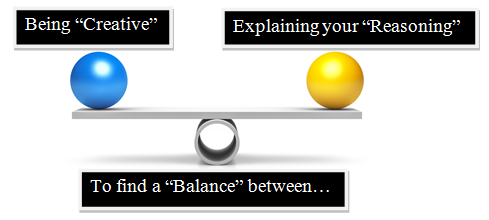# Jep - Geometry!

11 Questions | Total Attempts: 1142SettingsThink back to the "IDEAS" and "GEOMETRY" you used to complete your JEP. The "minimum" passing grade is 100%. Good Luck :)

• 1.
These two lines are called _________ lines.
• 2.
The "red line" is ________ing the angle.
• 3.
Point C can be called the "Point of _____________"
• 4.
How many points of intersection are shown in the following diagram?
• A.

5

• B.

2

• C.

7

• D.

3

• 5.
We started the JEP by making 3 Assumptions about what was best for the 3 cities.Assumptions are important because they are "SMART GUESSES" which help us figure out where to start when solving a problem!!!
• A.

True

• B.

False

• 6.
What was the PURPOSE of our JEP(Junior Engineering Project)?Check ALL ANSWERS that you feel are correct!!!
• A.

To find a "balance" between being creative and being able to explain your reasoning

• B.

To understand the situation and figure out a solution using Geometry.

• C.

To drive your teacher crazy not following directions!!!

• D.

To learn new things about "solving a problem" using your own ideas and explaining them with Math.

• 7.
Point B is called the ____________.
• 8.
Does the diagram below describe your Assumption A or Assumption B airport location? The r (radius) is EQUAL DISTANCE from all three points on the circle.
• A.

Assumption A

• B.

Assumption B

• 9.
Does the diagram below describe your Assumption A or Assumption B airport location? The r (radius) is EQUAL DISTANCE from the center of the circle to all three sides.
• A.

Assumption A

• B.

Assumption B

• 10.
Which one do you like?
• A.

Option 1

• B.

Option 2

• C.

Option 3

• D.

Option 4

Related Topics